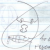## onionman

### Brandon joined Sep 30, 2010

VR mobile developer??

My Blogs

#### Billboarding with OpenGL

onionman Blog

Standard Billboards

So billboarding in games development was something that always used to frighten me. I understood you were supposed to make an object always face the screen, but I didn't know an easy way to do that. I mean, we're you supposed to somehow calculate all the rotations needed by glRotatef() to make a picture always face you?

Anyway, eventually I found a solution with some online help that made the whole thing really easy, and I couldn't find it anywhere else, so I just thought I'd share.

Basically, you just take the current rotation matrix and invert it. Pretty simple! So here's some sample code to do that.

``````	GLfloat modelview;
GLfloat tempModelView;
glGetFloatv( GL_MODELVIEW_MATRIX, modelview );
glGetFloatv( GL_MODELVIEW_MATRIX, tempModelView );

modelview = tempModelView;
modelview = tempModelView;
modelview = tempModelView;
modelview = 0.f;

modelview = tempModelView;
modelview = tempModelView;
modelview = tempModelView;
modelview = 0.f;

modelview = tempModelView;
modelview = tempModelView;
modelview = tempModelView;
modelview = 0.f;

//if you'd like, plug the position of the object into the transformation matrix, here I'm drawing a sun
modelview = sunPosition.position;
modelview = sunPosition.position;
modelview = sunPosition.position;
modelview = 1.f;

glPushMatrix();
glMultMatrixf(modelview);

renderFlare(0.8f,0.8f,0.6f,1.f,5.f); //render the billboard

glPopMatrix();
``````

Semi-Billboards: Lasers

So a friend also asked me how I drew the lasers in our upcoming game Ensign 1. These really aren't anything special, and still need some work. Still, the basic idea is that these objects should be drawn facing the direction they are traveling, but their up vector should be pointing into the line of site of the viewer. Iunno if I explained that right, but anyway, here's the code I used to do it.
Basically you generate the forward, up, and right vectors for this object. And you need to ensure that the up vector is completely orthogonal to the forward vector.

``````glDepthMask(GL_FALSE);
Vector up,forward,right;

forward = lasers[index].velocity;
forward = forward*6.f;

up = lasers[index].position - parentModel->position;
up -= forward * forward.dot(forward,up); //ensure up vector is orthogonal to forward vector
up.normalize();

right = up.cross(forward,up);
right.normalize();
right = right*1.f;

GLfloat modelView;

modelView = right;
modelView = right;
modelView = right;
modelView = 0.f;

modelView = up;
modelView = up;
modelView = up;
modelView = 0.f;

modelView = forward;
modelView = forward;
modelView = forward;
modelView = 0.f;

modelView = lasers[index].position;
modelView = lasers[index].position;
modelView = lasers[index].position;
modelView = 1.f;

glPushMatrix();
glMultMatrixf(modelView);

glEnable(GL_BLEND);
lasers[index].Draw(viewerPosition);
glDisable(GL_BLEND);
glPopMatrix();

``````

Some more tricks would might be that, when the up vector here is very small, the laser might appear flat, you might actually purposely add some forward vector to it to give it some depth. I haven't tried this myself yet though.

Anyway, enjoy!

Profile
62%
20
##### AvatarMale
Blog Statistics
1
1,309
0